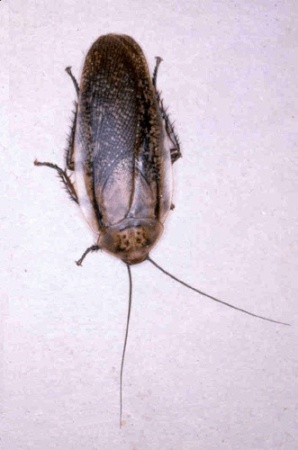Next Atypical Types 70

# Where's the Bug?

```        sort [] = []
sort ls = merge (sort p) (sort q)
where (p, q) = split ls
```Suppose the function is trying to sort a one-element list [x]

It calls split and gets ([x], [])

Then it tries to recursively sort the two parts

Sorting [] is okay.

Sorting [x] puts it into an infinite loop

 NextCopyright © 1999,2008 Mark Dominus